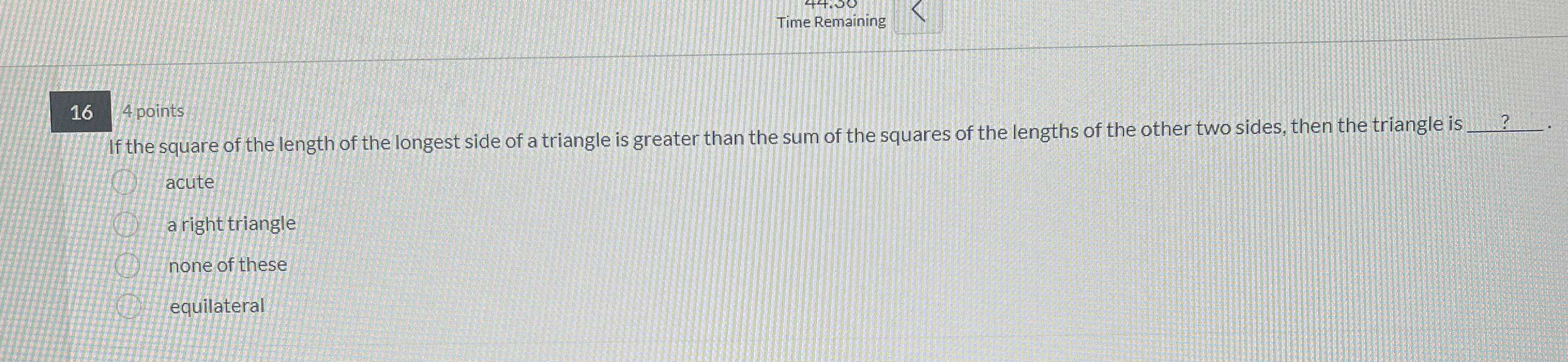### ¿Todavía tienes preguntas de matemáticas?

Pregunte a nuestros tutores expertos
Algebra
Pregunta$$16$$ points

If the square of the length of the longest side of a triangle is greater than the sum of the squares of the lengths of the other two sides, then the triangle is

acute

a right triangle

none of these

equilateral